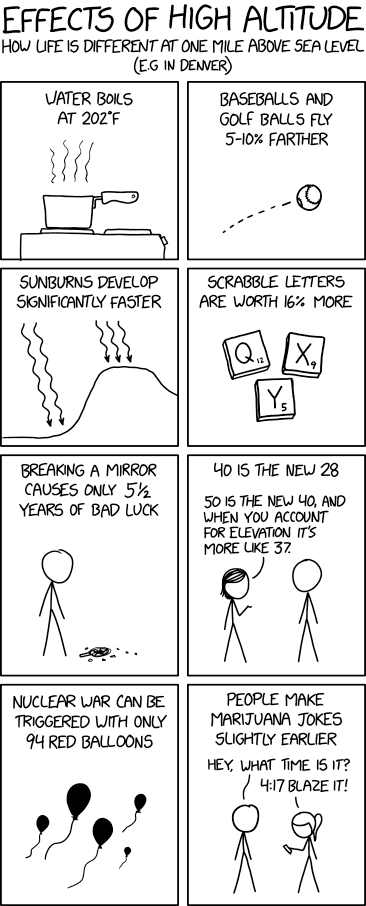assignment Homework

##### Bottle in a Bottle 2
heat entropy ideal gas Energy and Entropy 2021 (2 years)

Consider the bottle in a bottle problem in a previous problem set, summarized here.

A small bottle of helium is placed inside a large bottle, which otherwise contains vacuum. The inner bottle contains a slow leak, so that the helium leaks into the outer bottle. The inner bottle contains one tenth the volume of the outer bottle, which is insulated.

The volume of the small bottle is 0.001 m23 and the volume of the big bottle is 0.01 m3. The initial state of the gas in the small bottle was $p=106$ Pa and its temperature $T=300$ K. Approximate the helium gas as an ideal gas of equations of state $pV=Nk_BT$ and $U=\frac32 Nk_BT$.

1. How many molecules of gas does the large bottle contain? What is the final temperature of the gas?

2. Compute the integral $\int \frac{{\mathit{\unicode{273}}} Q}{T}$ and the change of entropy $\Delta S$ between the initial state (gas in the small bottle) and the final state (gas leaked in the big bottle).

3. Discuss your results.

60 min.

##### Free expansion
Energy and Entropy 2021 (2 years)

Students will determine the change in entropy (positive, negative, or none) for both the system and surroundings in three different cases. This is followed by an active whole-class discussion about where the entropy comes from during an irreversible process.

assignment Homework

##### Ideal gas in two dimensions
Ideal gas Entropy Chemical potential Thermal and Statistical Physics 2020
1. Find the chemical potential of an ideal monatomic gas in two dimensions, with $N$ atoms confined to a square of area $A=L^2$. The spin is zero.

2. Find an expression for the energy $U$ of the gas.

3. Find an expression for the entropy $\sigma$. The temperature is $kT$.

face Lecture

30 min.

##### Review of Thermal Physics
Thermal and Statistical Physics 2020

These are notes, essentially the equation sheet, from the final review session for Thermal and Statistical Physics.

face Lecture

120 min.

##### Ideal Gas
Thermal and Statistical Physics 2020

These notes from week 6 of Thermal and Statistical Physics cover the ideal gas from a grand canonical standpoint starting with the solutions to a particle in a three-dimensional box. They include a number of small group activities.

assignment Homework

##### Using Gibbs Free Energy
thermodynamics entropy heat capacity internal energy equation of state Energy and Entropy 2021 (2 years)

You are given the following Gibbs free energy: \begin{equation*} G=-k T N \ln \left(\frac{a T^{5 / 2}}{p}\right) \end{equation*} where $a$ is a constant (whose dimensions make the argument of the logarithm dimensionless).

1. Compute the entropy.

2. Work out the heat capacity at constant pressure $C_p$.

3. Find the connection among $V$, $p$, $N$, and $T$, which is called the equation of state (Hint: find the volume as a partial derivative of the Gibbs free energy).

4. Compute the internal energy $U$.

group Small Group Activity

30 min.

##### Heat and Temperature of Water Vapor

In this introduction to heat capacity, students determine a derivative that indicates how much the internal energy changes as the temperature changes when volume is held constant.

assignment Homework

##### One-dimensional gas
Ideal gas Entropy Tempurature Thermal and Statistical Physics 2020 Consider an ideal gas of $N$ particles, each of mass $M$, confined to a one-dimensional line of length $L$. The particles have spin zero (so you can ignore spin) and do not interact with one another. Find the entropy at temperature $T$. You may assume that the temperature is high enough that $k_B T$ is much greater than the ground state energy of one particle.

assignment Homework

##### Entropy, energy, and enthalpy of van der Waals gas
Van der Waals gas Enthalpy Entropy Thermal and Statistical Physics 2020

In this entire problem, keep results to first order in the van der Waals correction terms $a$ and \$b.

1. Show that the entropy of the van der Waals gas is \begin{align} S &= Nk\left\{\ln\left(\frac{n_Q(V-Nb)}{N}\right)+\frac52\right\} \end{align}

2. Show that the energy is \begin{align} U &= \frac32 NkT - \frac{N^2a}{V} \end{align}

3. Show that the enthalpy $H\equiv U+pV$ is \begin{align} H(T,V) &= \frac52NkT + \frac{N^2bkT}{V} - 2\frac{N^2a}{V} \\ H(T,p) &= \frac52NkT + Nbp - \frac{2Nap}{kT} \end{align}Effects of High Altitude by Randall Munroe, at xkcd.

assignment Homework

##### Entropy of mixing
Entropy Equilibrium Sackur-Tetrode Thermal and Statistical Physics 2020

Suppose that a system of $N$ atoms of type $A$ is placed in diffusive contact with a system of $N$ atoms of type $B$ at the same temperature and volume.

1. Show that after diffusive equilibrium is reached the total entropy is increased by $2Nk\ln 2$. The entropy increase $2Nk\ln 2$ is known as the entropy of mixing.

2. If the atoms are identical ($A=B$), show that there is no increase in entropy when diffusive contact is established. The difference has been called the Gibbs paradox.

3. Since the Helmholtz free energy is lower for the mixed $AB$ than for the separated $A$ and $B$, it should be possible to extract work from the mixing process. Construct a process that could extract work as the two gasses are mixed at fixed temperature. You will probably need to use walls that are permeable to one gas but not the other.

Note

This course has not yet covered work, but it was covered in Energy and Entropy, so you may need to stretch your memory to finish part (c).

face Lecture

120 min.

##### Phase transformations
Thermal and Statistical Physics 2020

These lecture notes from the ninth week of Thermal and Statistical Physics cover phase transformations, the Clausius-Clapeyron relation, mean field theory and more. They include a number of small group activities.

face Lecture

120 min.

##### Fermi and Bose gases
Thermal and Statistical Physics 2020

These lecture notes from week 7 of Thermal and Statistical Physics apply the grand canonical ensemble to fermion and bosons ideal gasses. They include a few small group activities.